6. **What Economists Have to Say About Innovation, and the Rate of Ideas Growth** ¶

**6.1. The Depressing Bottom Line** ¶

The rate of economic growth—first in population, and more recently in average living standards and productivity growth rates—hinges on the proportional rate of increase $h$ in the human stock of useful ideas $H$. And this rate has been extraordinarily variable in the long sweep of human history. And if the trends of the past century and a half ere to continue for trhe next three, we would look forward to truly cray-cray levels of abundant human wealth:Moreover, right now the divergences across national economies are as great as they have ever been, and orders of magnitude greater than they were three centuries ago or even one century ago. What insights can economists offer into these phenomena?

Unfortunately, the bottom line is that economists have little that is terribly useful to say about the proportional rate $h$ at which the human stock $H$ of useful and valuable ideas about technology and organization increases. It would not be too much a parody to say that economists know only four things:

1. People learn by doing: trying to produce, and succesfully producing, brings with it knowledge about how to produce more efficiently and effectively.
2. People learn by investing: a great deal of knowledge is embodied in the particular capital goods themselves produced and deployed; if you do not invest, a great deal of your knowledge remains theoretical.
3. People learn by researching and developing: focused attention on the process of developing technology can be very effective—much more so than simply relying on the side-effects of those whose major focus is on production itself
4. Knowledge is non-rival: once it is generated, it can and should be spread as widely as possible, for there is no downside for society as a whole from sharing.

But that—especially that without sound and solid quantitative estimates of the size and importance of these effects and channels—is rather thin gruel given that the growth and diffusion of useful knowledge about production and organization is the big enchilada in the process of economic growth.

Here we will focus on (3) and (4), leaving (1) and (2) for later "applications" sections of this course:

**6.2. Knowledge Is Non-Rival** ¶

**6.2.1. Logical Implications** ¶

Useful ideas about technology and organization are non-rival: one person's work in adding to $H$ can rapidly benefit all—if it is allowed to spread. And attempts to keep it from spreading—to limit knowledge's distribution by somehow charging those using it a price—must violate the optimality condition that the costs imposed on people for making use of commodities reflect and match the burden that their withdrawal of the commodities from the common stock imposes on the rest of the community, for with non-rival commodities there is no such withdrawal. The insights that knowledge is key and that knowledge is non-rival are now nearly two centuries old. We can find them in in 1843: Friedrich Engels (1843): Outlines of a Critique of Political Economy https://www.marxists.org/archive/marx/works/1844/df-jahrbucher/outlines.htm:

According to the economists, the production costs of a commodity consist of three elements: the rent for the piece of land required to produce the raw material; the capital with its profit, and the wages for the labour required for production and manufacture.... [Since] capital is “stored-up labour”... two sides–the natural, objective side, land; and the human, subjective side, labour, which includes capital and, besides capital, a third factor which the economist does not think about–I mean the mental element of invention, of thought, alongside the physical element of sheer labour.

What has the economist to do with inventiveness? Have not all inventions fallen into his lap without any effort on his part? Has one of them cost him anything? Why then should he bother about them in the calculation of production costs? Land, capital and labour are for him the conditions of wealth, and he requires nothing else. Science is no concern of his.

What does it matter to him that he has received its gifts through Berthollet, Davy, Liebig, Watt, Cartwright, etc.–gifts which have benefited him and his production immeasurably? He does not know how to calculate such things; the advances of science go beyond his figures. But in a rational order which has gone beyond the division of interests as it is found with the economist, the mental element certainly belongs among the elements of production and will find its place, too, in economics among the costs of production.

And here it is certainly gratifying to know that the promotion of science also brings its material reward; to know that a single achievement of science like James Watt’s steam-engine has brought in more for the world in the first fifty years of its existence than the world has spent on the promotion of science since the beginning of time...

And yet indeed it was the case that mainstream economists, for generations, paid remarkably little of their attention to "inventiveness". Engels was right—at least about mainstream economists' strange neglect. (I take no stance on whether Engels was right in his belief that mainstream economists cannot see the world as it is but only illusions that are caused by our particular institutional framework and convenient to those whom our current institutional framework serves most fulsomely.) Engels was right so much so that Paul Romer received the Nobel Prize in 2018 for his attempts to bring "inventiveness" back to the center. When Robert Solow (1987): Growth Theory and After https://www.nobelprize.org/prizes/economic-sciences/1987/solow/lecture/ gave his Nobel lecture on the occasion of the earlier "economic growth" Nobel Prize, awarded in 1987, he noted that his theory had little to say about “technical change in the broadest sense” and that this was a huge flaw:

The “neoclassical model of economic growth” started a small industry... stimulated hundreds of theoretical and empirical articles... very quickly found its way into textbooks... is what allows me to think that I am a respectable person to be giving this lecture today.... Gross output per hour of work in the U. S. economy doubled between 1909 and 1949; and some seven-eighths of that increase could be attributed to “technical change in the broadest sense” and only the remaining eight could be attributed to conventional increase in capital intensity.... I had expected to find a larger role for straightforward capital formation...

Solow goes on to write that his attempts to say something meaningful and important about the determinants of $h$ failed:

"embodiment", the fact that much technological progress, maybe most of it, could find its way into actual production only with the use of new and different capital equipment... [and so] a policy to increase investment would thus lead... also to a faster transfer of new technology into actual production, which would [matter much].... That idea seemed to correspond to common sense, and it still does.... If common sense was right, the embodiment model should have fit the facts significantly better than the earlier one. But it did not...

There is a literature, springing from Paul Romer's work in the 1980s, focusing on the implications of non-rivalry in the use of ideas: that one person's work in adding to $H$ can rapidly benefit all. The first conclusion is that production must in some sense be subject to increasing returns. We know that if all material "inputs" were to double then, since the new inputs could just do the same things as the old ones, production would at least double. In knowledge production, however, pursuing the same lines of inquiry and thus making all the same discoveries twice would be silly. Doubled material inputs with doubled effort devoted to knowledge creation should therefore more than double output. An economy in which non-rival knowledge is important will therefore exhibit scale effects: size matters, and in a good way for growth.

The useful literature can be seen as building on this first conclusion.

**6.2.2. Fitting the Entire Span of Human History: Michael Kremer on Growth since One Million B.C.**¶

**6.2.2.1. Theory**: Non-rivalry in the use of and non-crowding in the production of useful ideas about technology and organization are the fundamental underlying assumptions of the first milestone to visit on our path through the literature: Michael Kremer: Michael Kremer (1993) Population Growth and Technological Change: One Million B.C. to 1990 https://delong.typepad.com/files/kremer-million.pdf:

The long-run history of population growth and technological change is consistent with the population implications of models of endogenous technological change... a highly stylized model in which... the growth rate of technology is proportional to total population... the Malthusian assumption that population is limited by the available technology, so that the growth rate of population is proportional to the growth rate of technology. Combining these assumptions implies that the growth rate of population is proportional to the level of population.... The prediction that the population growth rate will be proportional to the level of population is broadly consistent with the data... until recently.... If population grows at finite speed when income is above its steady state... per capita income will rise over time. If population growth declines in income at high levels of income, as is consistent with a variety of theoretical models and with the empirical evidence, this gradual increase in income will eventually lead to a decline in population growth.... As the model predicts, the growth rate of population has been propor- tional to its level over most of history.... Among technologically separate societies, those with higher initial population had faster growth rates of technology and population...

In short, two heads are better than one:

Assume that output is given by

$Y = Ap^\alpha R^{1-\alpha}$

where A is the level of technology, p is population, and $R = 1$ is land, henceforth normalized to one. Per capita income $y$ therefore equals $Ap^{\alpha - 1}$. opulation increases above the steady-state equilibrium level of per capita income $y^*$ and decreases below it. Diminishing returns to labor imply that a unique level of population, $p^*$ generates income $y^*$:

$p^* = \left( \frac{A}{y^*} \right)^{(1/(1-\alpha))}$

In a larger population there will be proportionally more people lucky or smart enough to come up with new ideas. If research productivity per person is independent of population and if A affects research output the same way it affects output of goods (linearly, by definition), then the rate of change of technology will be:

$\frac{dA}{dt} = \pi Ap$

Take the log derivative of the population determination equation:

$\frac{dln(p)}{dt} = \left( \frac{1}{1-\alpha} \right) \frac{dln(A)}{dt}$

and substitute in the expression for the growth rate of technology:

$\frac{dp}{dt} = \frac{\pi p^2}{1-\alpha}$

to get superexponential growth of population (and total income)—as long as the Malthusian régime lasts, there is no demographic transition, .

To get an idea of what this means, let us run a computational experiment. There were 2.5 million people 10,000 years ago, at the invention of agriculture. There were 15 million people 5,000 years ago, at the invention of writing. There were 170 million people in the year 1. Let's calibrate this model to 2.5 million people in the year -8000 and 15 million people in the year -3000: a value of $π/(1-α) = 0.00006666$ serves. But that value predicts that human population would cross 170 million heading upwards not in the year 1 but in the year -2080: early in the Bronze Age.

In :
# fitting the Kremer model to historical population
# for years -8000 and -3000:

import numpy as np
import pandas as pd
import matplotlib.pyplot as plt
%matplotlib inline

Year = [-8000]
P = [2.5]
α = 0.5
π = 0.00003333

for t in range(-7999, -2080):
Year = Year + [t]
P = P + [P[t+7999] + π*P[t+7999]**2/(1-α)]

List = [Year, P]
P_df = pd.DataFrame(List).transpose()
P_df['Log Population'] = np.log(P)
nlabel = ['Year', 'Population', 'Log_Population']
P_df.columns=nlabel
P_df.set_index('Year', inplace = True)

P_df.Population.plot()

plt.ylabel('Population')
plt.xlabel('Years')
plt.title(' Human Population')
plt.show()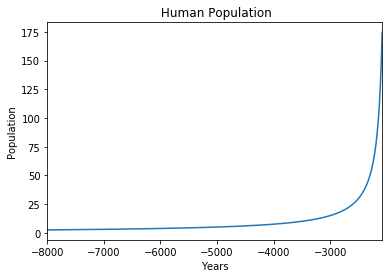If we want to fit our three pre-1 benchmarks, we cannot have two heads being fully as good as one. So, instead, let us assume not that a 1% increase in the STEM workforce raises the rate of technological progress by 1% but rather by λ% for some parameter λ. So the dynamics for population then become:

$\frac{dp}{dt} = \frac{\pi p^{1+\lambda}}{1-\alpha}$

$α = 0.5$, $π = 0.00003264$, $λ = 0.8529$ fit the pre-1 benchmarks well. But those benchmarks predict that the human population would have exploded in the following two centuries, and crossed rthe world's current population of 7.6 billion in the year 221.

Even if two heads are not quite as good as one—are only 1.85 times as good as one—there need to be other sources of drag in order to have kept the world from an Industrial Revolution-class breakthrough late in the Later Han, and under the late Antonine and Severian dynasties:

In :
# fitting the Kremer model to historical population
# for years -8000, -3000, and 1:

import numpy as np
import pandas as pd
import matplotlib.pyplot as plt
%matplotlib inline

Year = [-8000]
P = [2.5]
α = 0.5
π = 0.00003264
λ = 0.8529

for t in range(-7999, 221):
Year = Year + [t]
P = P + [P[t+7999] + π*P[t+7999]**(1+λ)/(1-α)]

List = [Year, P]
P_df = pd.DataFrame(List).transpose()
P_df['Log Population'] = np.log(P)
nlabel = ['Year', 'Population', 'Log_Population']
P_df.columns=nlabel
P_df.set_index('Year', inplace = True)

P_df.Population.plot()

plt.ylabel('Population')
plt.xlabel('Years')
plt.title(' Human Population')
plt.show()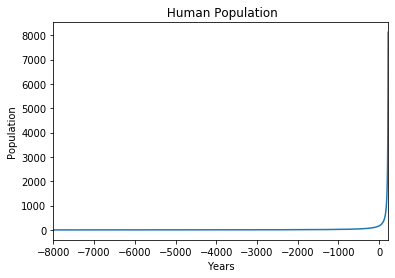**6.2.2.2. How Well Does This Fit Human History?**: Still, all in all, Michael Kremer says: it does not fit badly badly. And, indeed, up to 1900 the rate of change of the human population is indeed roughly proportional to the square of the population—as long as we start not with the invention of agriculture but with the invention of writing, and with a hiccup as the Roman and Han empires collapsed in the second third of the first millennium. After 1900 things fall apart: increasing populations and the increasing ability of people to use technology and wealth to help their investigations do not pay dividends, either in further accelerating population growth or in increasing the rate of growth of global incomes.Nevertheless, two heads are better than one. Or maybe not: surely the effective STEM labor force depends on means of knowledge recording and communication. And it is not foolish to expect ex ante that there would be some diminishing returns from exhaustion of low-hanging fruit at some point. We do seem to see a jump up in growth with the invention of writing, and cities. Shouldn't we also see a jump up with the alphabet? Shouldn't we also see a jump up with the invention of printing? Perhaps the effects of the picking of the low-hanging fruit in exhausting opportunities and slowing growth civilization-wide are visible in the slowdown after the year one. Perhaps the effective STEM labor force gets big bumps up with the alphabet and with printing that together, in the large, offset this exhaustion.

Clearly, however, two heads are better than one will not suffice to understand the relative constancy of global economic growth rates since the coming of modern economic growth around 1870, or even the failure of Roman and Han civilization to usher in an industrial revolution.

**6.2.3. Chad Jones on R & D-Based Models of Economic Growth** ¶

Can we preserve the insights that ideas are non-rival and that technology is the ballgame and still understand why growth did not accelerate faster and bring us an Industrial Revolution early in the first millennium, and, in fact, has not further accelerated since the late 1800s? Chad Jones believes we can, and he lays out his case in Charles I. Jones (1995): R&D-Based Models of Economic Growth https://delong.typepad.com/files/jones-r--d.pdf:

The prediction of permanent scale effects on growth from the R&D equation means that the models of Romer/Grossman-Helpman/Aghion-Howitt and others are all easily rejected.... However, the R&D-based models [remain] intuitively very appealing.... [Is there] a way to maintain the basic structure of these models while eliminating the prediction of [permanent] scale effects [on the rate of growth?]...

Jones's answer is "yes". Jones accomplishes this by building a basic model that has both (a) an "as the low-hanging technological fruit is picked, maintaining the same proportional growth rate for the ideas stock $H$ becomes harder" effect (the parameter $\phi < 1$); and (b) an "as the STEM workforce increases, researchers tend to step on each others' toes and get in each others' way" effect (the parameter $\lambda < 1$):

$\frac{dH}{dt} = \pi L_{stem}^{\lambda} H^{\phi}$

$\frac{dH/dt}{H} = \pi L_{stem}^{\lambda} H^{\phi - 1}$

To gain some intuition, let's consider six different economies in which the rate of growth $n$ of the STEM labor force varies from 0 to 6% per year, in which the initial levels of both the ideas stock $H_0$ and the STEM labor force $L_0$ are set at 1, and let us set the R&D crowding parameter $\lambda = 0.5$ and, just to get striking results, the exhaustion of low hanging fruit parameter at the very low level of $\phi = 0.1$. Looking out at the evolution of the log of the ideas stock for 400 years:

In :
# watching how H evolves for different values
# of STEM labor force growth, from 0 to 5%
# per year

import numpy as np
import pandas as pd
import matplotlib.pyplot as plt
%matplotlib inline

T = 400
δ = 0.1
H_0 = 1
n = [0, 0.01, 0.02, 0.03, 0.04, 0.05]
nlabel = ['0%', '1%', '2%', '3%', '4%', '5%']
φ = 0.1
λ = 0.5
L_0 = 1
J = []

for i in range(6):
H = [H_0]
for t in range(T):
H = H + [H[t]+(δ*H[t]**φ)*(L_0**λ)*np.exp(λ*n[i]*t)]
J = J + [H]

J_df = pd.DataFrame(J)

Jones_df = J_df.transpose()

Jones_df.columns=nlabel

np.log(Jones_df).plot()

plt.ylabel('Log Ideas Stock')
plt.xlabel('Years')
plt.title('Log of Ideas Stock for n Between 0 and 6% per Year')
plt.show()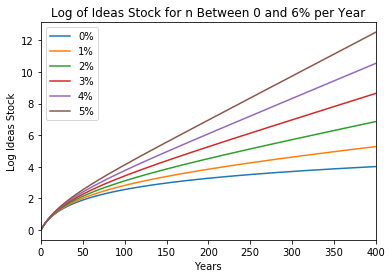What is going on here? We can see from the constancy of the slopes on the right hand side of this log graph that the ideas stock $H$ is heading for some steady-state growth rate that is higher the higher is the rate of growth of the STEM labor force. And for that convergence to a constant growth rate to happen, in the long run the increase in the effective STEM labor force $L_{STEM}^{\lambda}$ has to be exactly offset by diminishing returns to innovative effort $\delta H^{\phi - 1}$. Thus along the ideas-stock steady-state balanced-growth path:

$\lambda \frac{1}{L_{stem}} \frac{dL_{stem}}{dt} = (1 - \phi) \frac{dH/dt}{H}$

$\lambda n_{stem} = (1 - \phi) h^*$

The level of ideas $H^*$ at which that growth rate $h^*$ would be attained is characterized by:

$\frac{\lambda n_{stem}}{1 - \phi} = \pi L_{stem}^{\lambda} (H^*)^{\phi-1}$

So $H$ grows more rapidly than $h^*$ until it closes in on the value of $H^*$ that is on the steady-state balanced-growth path:

$H^* =$ $\left( \frac{\pi (1 - \phi)}{\lambda} \right)^{1/(1-\phi)}$ $\left( \frac{1}{n_{stem}} \right)^{1/(1-\phi)}$ $L_{stem}^{\lambda/(1-\phi)}$

And then the growth rate in the steady-state is characterized by:

$h^* = \left( \frac{\lambda}{1 - \phi} \right) n_{stem}$

Thus the rate of growth of the ideas stock along the steady-state balanced-growth growth path will be proportional to the rate of growth of the STEM labor force with constant of proportionality $\lambda / (1 - \phi)$ (the degree to which more researchers step on one anothers' toes divided by how important it is that the low-hanging innovation fruit has already been picked), and the level of the ideas stock along the steady-state growth path will vary inversely with the rate of growth $n$ of the STEM labor force raised to the power ${1/(1-\phi)}$, and directly with the level $L_{stem}$ of the STEM labor force raised to the power ${\lambda/(1-\phi)}$.

If $H < H^*$, then $H$ is growing faster than $h^*$, and the scale variable $H L_{stem}^{-\lambda/(1-\phi)}$ is rising. When $H = H^*$, then $h = h^*$, and the scale variable is then constant:

$H L_{stem}^{-\lambda/(1-\phi)} = \left( \pi (1 - \phi)/\lambda \right)^{1/(1-\phi)} n_{stem}^{-1/(1-\phi)}$

And, indeed, looking at the levels of the ideas stock over 150 years reveals, first, initial superexponential growth; that growth rate then declines until the growth rate asymptotes (for $n > 0$) to merely exponential growth at the rate $h^* = \lambda n_{stem} / (1 - \phi )$ :

In :
# watching how H evolves for different values
# of STEM labor force growth, from 0 to 5%
# per year

import numpy as np
import pandas as pd
import matplotlib.pyplot as plt
%matplotlib inline

T = 250
π = 0.01
H_0 = 10
n_stem = [0, 0.01, 0.02, 0.03, 0.04, 0.05]
nlabel = ['0%', '1%', '2%', '3%', '4%', '5%']
φ = 0.1
λ = 0.5
L_0 = 1
J = []

for i in range(6):
H = [H_0]
for t in range(T):
H = H + [H[t]+(π*H[t]**φ)*(L_0**λ)*np.exp(λ*n_{stem}[i]*t)]
J = J + [H]

J_df = pd.DataFrame(J)

Jones_df = J_df.transpose()

Jones_df.columns=nlabel

Jones_df.plot()

plt.ylabel('Ideas Stock')
plt.xlabel('Years')
plt.title('Ideas Stock for n Between 0 and 6% per Year')
plt.show()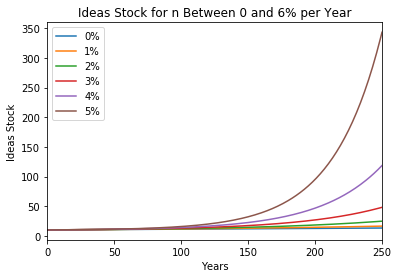How fast does this Jones model converge to its steady-state balanced-growth path with its constant rate of increase $h^*$ in the ideas stock? To understand this, we need to look at our scale variable $H L_{stem}^{-\lambda/(1-\phi)}$:

In :
# watching how the scale variable evolves for different values
# of STEM labor force growth, from 0 to 5% per year

import numpy as np
import pandas as pd
import matplotlib.pyplot as plt
%matplotlib inline

T = 500
π = 0.01
H_0 = 10
n_{stem} = [0.005, 0.01, 0.02, 0.03, 0.04, 0.05]
nlabel = ['H_half', 'L_half', 'H_1', 'L_1', 'H_2', 'L_2', 'H_3', 'L_3', 'H_4', 'L_4', 'H_5', 'L_5']
φ = 0.5
λ = 0.5
L_0 = 1
J = []

for i in range(6):
H = [H_0]
L = [L_0]
for t in range(T):
H = H + [H[t]+(π*H[t]**φ*(L_0**λ)*(np.exp(λ*n_{stem}[i]*t)))]
L = L +[L[t]*np.exp(n[i])]
J = J + [H]
J = J + [L]

J_df = pd.DataFrame(J)

Jones_df = J_df.transpose()

Jones_df.columns=nlabel

Jones_df['Sc_half'] = Jones_df.H_half*(Jones_df.L_half**(-λ/(1-φ)))
Jones_df['Sc_1'] = Jones_df.H_1*(Jones_df.L_1**(-λ/(1-φ)))
Jones_df['Sc_2'] = Jones_df.H_2*(Jones_df.L_2**(-λ/(1-φ)))
Jones_df['Sc_3'] = Jones_df.H_3*(Jones_df.L_3**(-λ/(1-φ)))
Jones_df['Sc_4'] = Jones_df.H_4*(Jones_df.L_4**(-λ/(1-φ)))
Jones_df['Sc_5'] = Jones_df.H_5*(Jones_df.L_5**(-λ/(1-φ)))

sclabel = ['Sc_half', 'Sc_1', 'Sc_2', 'Sc_3', 'Sc_4', 'Sc_5']

Jones_df[sclabel].plot()

plt.ylabel('Scale Variable')
plt.xlabel('Years')
plt.title('Scale Variable for n Between 0.5% and 5% per Year')
plt.show()

plt.show()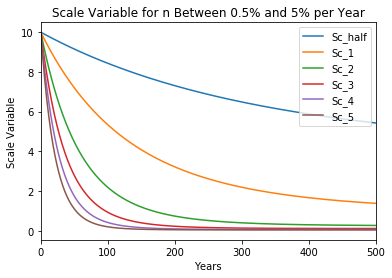In :
Jones_df['Div_Sc_half'] = Jones_df['Sc_half'] - 4
Jones_df['Div_Sc_1'] = Jones_df['Sc_1'] - 1
Jones_df['Div_Sc_2'] = Jones_df['Sc_2'] - 1/4
Jones_df['Div_Sc_3'] = Jones_df['Sc_3'] - 1/9
Jones_df['Div_Sc_4'] = Jones_df['Sc_4'] - 1/16
Jones_df['Div_Sc_5'] = Jones_df['Sc_5'] - 1/25

asymplabel = ['Div_Sc_half', 'Div_Sc_1', 'Div_Sc_2', 'Div_Sc_3', 'Div_Sc_4', 'Div_Sc_5']

np.log(Jones_df[asymplabel]).plot()

plt.ylabel('Log Scale Variable Divergence')
plt.xlabel('Years')
plt.title('Log Scale Variable Divergence for n Between 0.5% and 5% per Year')

plt.show()
/Users/delong1/opt/anaconda3/lib/python3.7/site-packages/ipykernel_launcher.py:10: RuntimeWarning: invalid value encountered in log
# Remove the CWD from sys.path while we load stuff.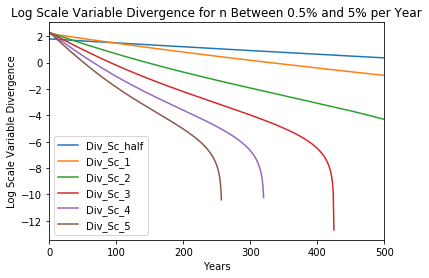**TO BE DONE: 6.2.4. Start with the Jones Rather than the Kremer Equation** ¶

$\frac{dH_t}{dt} = \pi H_t^{\phi} L^{\lambda}$

And consider how they would operate back in the Malthusian régime, in which the workforce is near subsistence and increases with ideas as:

$L = c_1 H^\gamma$

$\frac{dH}{dt} = \pi_{mal} c_1 H^{\phi + \gamma \lambda}$

After the Malthusian régime ends, if the population were to then become constant, we would find a shift to:

$\frac{dH}{dt} = \pi_{post} c_2 H^{\phi}$

Notes and Musings: Growth of the STEM Labor Force:

25 bachelor's degrees per 1000 23 year olds in 1900...

300 bachelor's degrees per 1000 23 year olds today...

60-fold multiplication in college graduates in the U.S....

20-fold multiplication in h since 1870

$\frac{\lambda}{1-\phi} = \frac{1}{3}$

45,000,000

Inflection points in the effective STEM workforce

• writing
• printing
• formal education
In :
# MEMO: dependence of H* on φ
#

φ = 0.0
λ = 0.5
n = 0.01
L_STEM = 1
π = 0.01
H_star_list = []
φ_list = []

for i in range (9):
φ = φ + 0.1
H_star = (π*(1-φ)/(λ*n))**(1/(1-φ))
H_star_list = H_star_list + [H_star]
φ_list = φ_list + [φ]

D = [φ_list,H_star_list]
Dep_df  = pd.DataFrame(D).transpose()
deplabel = ['φ', 'H*']

Dep_df.columns=deplabel

Dep_df.set_index('φ', inplace = True)

Dep_df
Out:
H*
φ
0.1 1.921481e+00
0.2 1.799492e+00
0.3 1.617165e+00
0.4 1.355092e+00
0.5 1.000000e+00
0.6 5.724334e-01
0.7 1.821815e-01
0.8 1.024000e-02
0.9 1.024000e-07

**Lecture Notes: What Economists Have to Say About the Rate of Ideas Growth** ¶